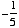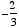index: click on a letter A B C D E F G H I J K L M N O P Q R S T U V W X Y Z A to Z index index: subject areas numbers & symbols sets, logic, proofs geometry algebra trigonometry advanced algebra & pre-calculus calculus advanced topics probability & statistics real world applications multimedia entrieswww.mathwords.com about mathwords website feedback

 Negative Reciprocal Opposite Reciprocal The result of taking the reciprocal of a number and then changing the sign. For example, the negative reciprocal of 5 is, and the negative reciprocal ofis. Note: Perpendicular lines have slopes that are negative reciprocals of each other.